# Area of a triangle: formula and how to find the area

Learn how to calculate the area of a triangle, explore their properties and have a go at some questions!Author
Sumaya Fodey

Last updated: July 19th, 2023# Area of a triangle

Learn how to calculate the area of a triangle, explore their properties and have a go at some questions!Author
Sumaya Fodey

Last updated: July 19th, 2023# Area of a triangle

Learn how to calculate the area of a triangle, explore their properties and have a go at some questions!Author
Sumaya Fodey

Last updated: July 19th, 2023

# Area of a triangle

Learn how to calculate the area of a triangle, explore their properties and have a go at some questions!Author
Sumaya Fodey

Last updated: July 19th, 2023

Many people feel frightened when they hear the word ‘geometry’. But fear no more! Whilst geometry can seem a little scary, it can actually be very fun – as long as you understand some basic formulas and how to use them to solve questions.

Triangles are a fundamental shape in geometry, and understanding their properties is really important for various fields of study. One such property is being able to calculate the area of a triangle.

Working out the area of a triangle isn’t quite as easy as working out the area of a square or a rectangle. It requires a little more thinking, but once you understand the formula, it’s not as tricky as you may think!

## What is 'area' in maths?

Before we can calculate the area of a triangle, it’s firstly important to understand what an ‘area’ even is!

An area in maths is the entire space taken up by a 2D shape.

The area of a triangle is the space enclosed by its 3 sides. The unit for area is in square units, such as m2. Every 2D shape, such as parallelogram, a rhombus, a square and a rectangle, has an area.

When working out the area of a 2D shape, it’s important to know the width and height of the shape, as this is what you’ll be using to calculate their area.

For example, the area of a square is one length squared. The reason for this is because all four sides of a  square has equal lengths. Whereas an area of a rectangle is the length x width!

### What are the different types of triangles?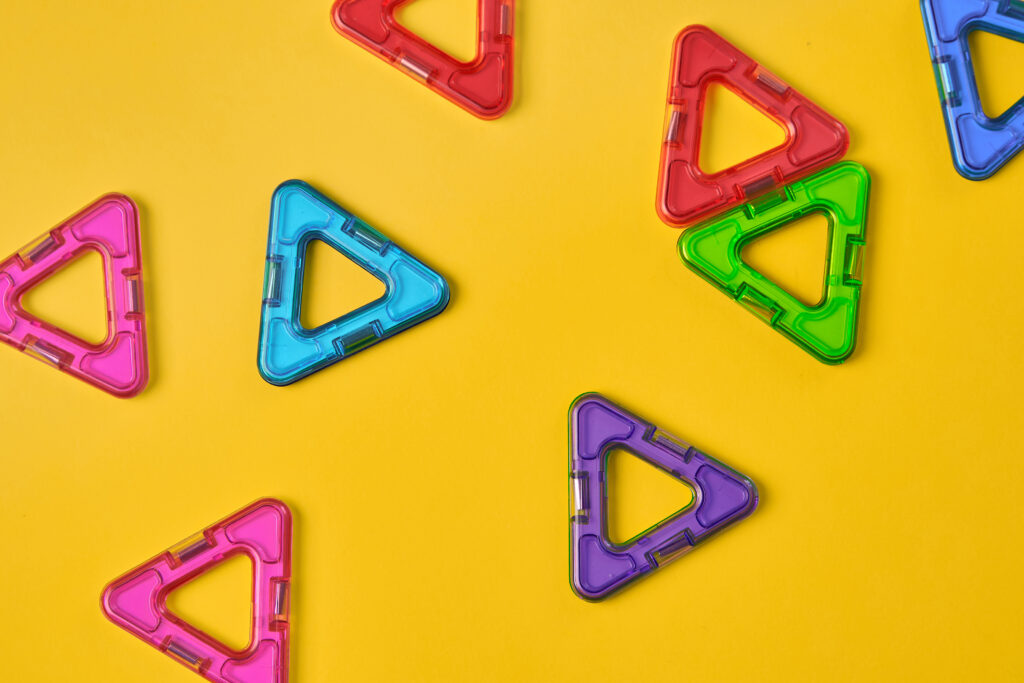Before you can calculate the area of a triangle, it’s important to know the different types of triangles. There are four types of triangles, and each has slightly different properties.

Scalene triangle

A scalene triangle has three sides that are all completely different lengths!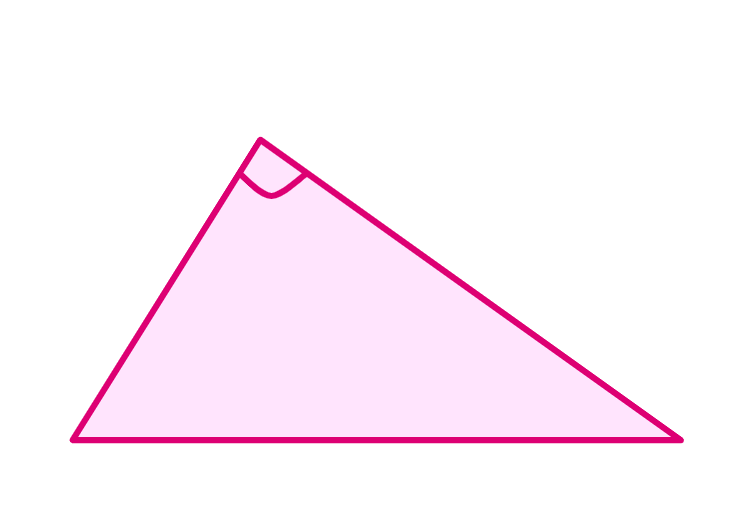Isosceles triangle

An isosceles triangle differs from scalene as two of its side are identical (the same length). This can be useful to keep in mind as you’ll be able to work out the length of one side if you know the other identical sides’ length.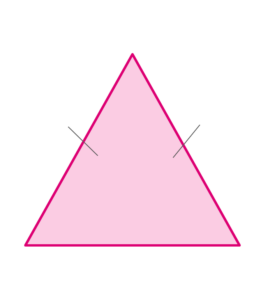Equilateral triangle

In an equilateral triangle, all three of its sides are equal (have exactly the same length). If you know the length of one side, you’ll know the length of the remaining sides! This can be helpful when working out geometric questions.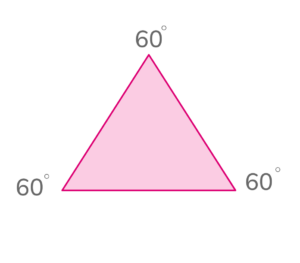Right-angled triangle

The name of this triangle is a giveaway as to what its defining feature is! To call a triangle right-angled, it has to have one angle that is 90 degrees. This triangle can be both scalene or even isosceles, as long as it has an angle that’s 90 degrees.

The reason a right-angled triangle cannot be an equilateral triangle is because the angles of an equilateral triangles are the same. All the angles of a triangle add up to 180 degrees, meaning each angle for an equilateral triangle is 60 degrees.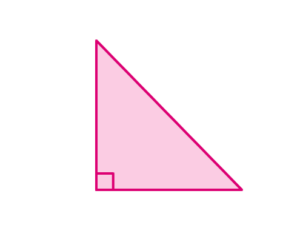## Explore triangles and area with DoodleMathsLooking for ways to bring triangles and shape to life? If so, DoodleMaths is here to help!

Created by our team of teachers, DoodleMaths is an award-winning app that’s filled with interactive questions and games that explore the whole primary curriculum.

Designed to be used ‘little and often’, it creates every child a unique learning experience tailored to their needs, boosting their confidence and ability in maths. And best of all, you can try it for free!### How to work out area of a triangle

Working out the area of a triangle is simpler than you may think. As long as you understand and can implement the formula, working out the area is easy!

The formula for working out the area of a triangle is:

= 1/2 x b x h    or   A = (b x h)/2     (both the same formula, written differently)

In this formula…

A = area

b = base

h = height

Now we know what the forumula is, let’s take a look at some practice questions!

### Practice questions: working out the area of a triangle

Question 1

Calculate the area of the triangle below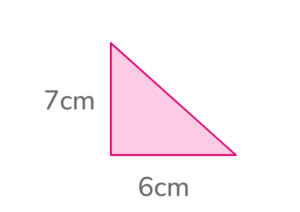Area= ½ x base x height

Area= ½ x 6 x 7 = 21 cm2

Question 2

What’s the area of the triangle below?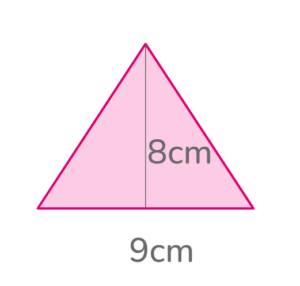Area = ½ x base x height

A= ½ x 9 x 8 = 36cm2

Question 3

Salma says the area of the triangle below is 35cm2. Do you agree with her? Explain your answer.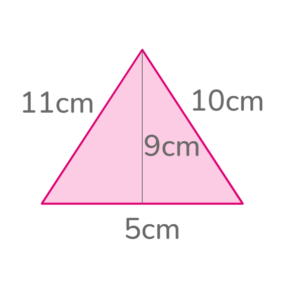A= ½ x base x height

Therefore A= ½ x 5 x 9

A= 22.50 cm2

So Salma is incorrect, because the area is 22.5cm2.

Question 4

Samantha deduced that the area of this triangle is less than 40cm2. Is she correct?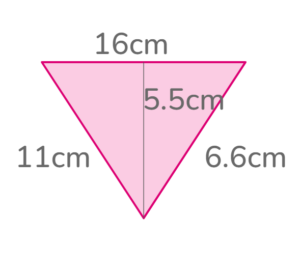She’s incorrect because A= ½ x 16 x 5.5 = 44 cm2

As you can see, calculating the area of a triangle isn’t as daunting as it seems! As long as you memorise and understand the formula, working out the area isn’t that difficult.

There are times when you’ll be given an irregular/different geometric shape and asked to work out the area. You can split the shape into triangles and then use the triangle formula to work out the area.

At first glance it will seem impossible, but it’s not any different to the examples above! Question 5 is an example of this.

Question 5

Calculate the area of the shape below.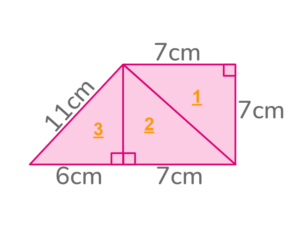We can start by splitting the shape into 3 triangles, as shown on the diagram by the orange numbers. We can then use other geometric shapes present, like the qualities of a square. Below is the working out.

Triangle 1: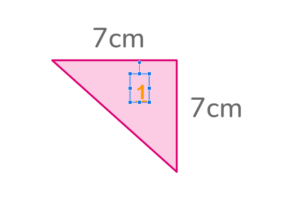Area= ½ x 7 x 7= 24.50cm2

We can work out the height of triangle 2 by remembering the qualities of a square.

Triangle 2: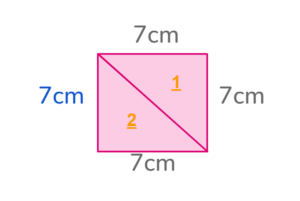The height of triangle 2 is 7cm, (shown in blue) as the sides of all squares are equal.

Therefore the area of triangle 2 =½ x 7 x 7= 24.50cm2

Triangle 3: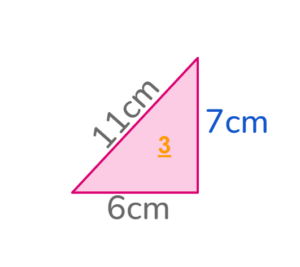Area of triangle 3= ½ x 6 x 7= 21cm2

Total area of the given shape= area of triangle 1 + area of triangle 2 + area of triangle 3

Total area= 24.50cm2+ 24.50cm2+ 21cm= 70cm2

## In summary...

With this triangle formula in mind, you can work out the area of any triangle – whether it’s scalene or isosceles!

If you’re looking for even more ways to explore shapes, DoodleMaths has you covered.

With interactive activities to enjoy, educational games to play and rewards to unlock, your child will always look forward to maths practice – and you can try it for free!Meet DoodleMaths, the app that's proven to boost ability in maths!# Are you a parent, teacher or student?

Are you a parent or teacher?

## Hi there!

Book a chat with our team